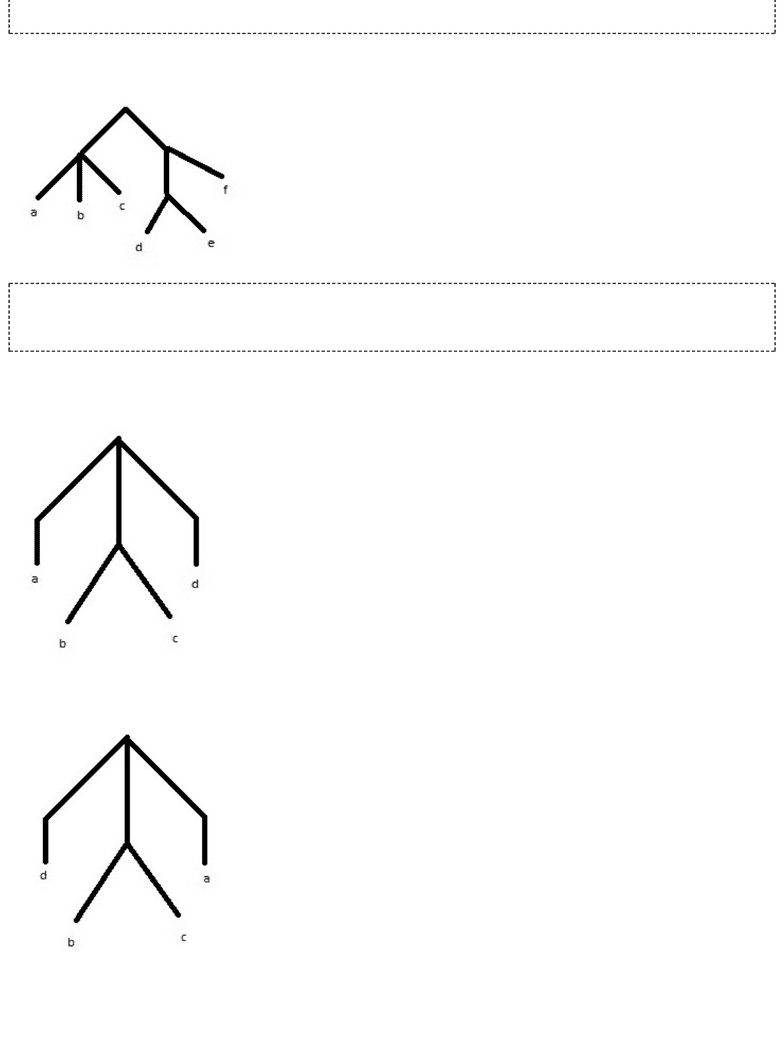Study Guides (390,000)
US (220,000)
Purdue (3,000)
CS (100)
All (10)
Midterm

# CS 17700 Study Guide - Midterm Guide: ViolExam

Department
Computer Sciences
Course Code
CS 17700
Professor
All
Study Guide
Midterm

This preview shows pages 1-3. to view the full 9 pages of the document.Midterm 2
Q1. What is the output of the following function?
def func1():
A = [4* for i in range(3)]
B = [3* for i in range(4)]
C = [3* for i in range(3)]
for i in range(3):
for j in range(3):
for k in range(4):
C[i][j] = C[i][j] + ( A[i][k] * B[k][j])
print C
(A) [[16,16,16], [16,16,16], [16,16,16]]
(B) *[[32,32,32], [32,32,32], [32,32,32]]
(C) [[8,8,8], [8,8,8], [8,8,8]]
(D) [[24,24,24], [24,24,24], [24,24,24]]
Q2. What is the output of the following function?
def func2():
m = [[1,1,1,1], [1,1,1,1], [1,1,1,1], [1,1,1,1]]
a = len(m)
b = len(m)
for j in range(b):
m[j] = 3
for k in range(a):
m[k][k] = 6
print m
(A) [[6, 0, 1, 1], [3, 6, 0, 1], [0, 6, 3, 1], [1, 6, 3, 0]]
(B) [[0, 1, 1, 6], [6, 0, 1, 3], [1, 0, 6, 1], [1, 1, 6, 3]]
(C) [[3, 3, 1, 1], [1, 3, 3, 1], [1, 1, 3, 3], [1, 3, 1, 3]]
(D) *[[6, 1, 1, 1], [3, 6, 1, 1], [3, 1, 6, 1], [3, 1, 1, 6]]
Q3. Suppose we have a large data matrix m, populated with values in the range 0-255. What is the output
of the following function?
def func4(m):
rows = len(m)
columns = len(m)
canvas = makeEmptyPicture(columns, rows, black)
show(canvas)
for row in range(rows):
for col in range(columns):
color = makeColor (m[row][col], m[row][col], m[row][col])
setColor(getPixel(canvas, col, row), color)
repaint(canvas)
(A) *A gray scale picture.
(B) A red scale picture.
(C) A green scale picture.
(D) A blue scale picture.
Q4. You are given the following code which prints the matrix m row by row:
def fun(m):
a = len(m)
b = len(m)
for i in range(b):

Only pages 1-3 are available for preview. Some parts have been intentionally blurred.for j in range(a):
print m[j][i]
m = [[1, 2, 3], [4,5,6]]
fun(m)
Which of the following functions prints the matrix column by column?
(i)
def fun(m):
a = len(m)
b = len(m)
for j in range(a):
for i in range(b):
print m[j][i]
(ii)
def fun(m):
a = len(m)
b = len(m)
for j in range(a):
for i in range(b):
print m[i][j]
(iii)
def fun(m):
a = len(m)
b = len(m)
for i in range(b):
for j in range(a):
print m[i][j]
(A) *i)
(B) ii)
(C) iii)
(D) i) and iii)
Q5.Consider the following tree:
tree = [["a","b", ["a","b"]],["a","b"],[["a","b"],"a"]]
Which of the following expressions resolves to “b”?
(i)
tree
(ii)
tree
(iii)
tree
(iv)
tree
(A) only i
(B) only iv

Only pages 1-3 are available for preview. Some parts have been intentionally blurred.(C) only iii
(D) *i, ii, and iii
Q6. Which list describes the following tree?
(A) [["a","b","c"],["d","e","f"]]
(B) [["a","b","c"],[["d","e"],["f"]]]
(C) *[["a","b","c"],[["d","e"],"f"]]
(D) none of the above
Q7. Which of the following trees has the value 'a' at index ?
i)
ii)
iii)